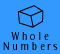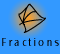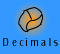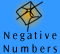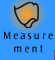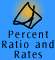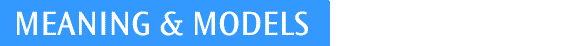| Place Value |
| Models for place value | Quick quiz |

Place Value

Our number system uses 10 digits to generate all the numbers we need. They are:

0 1 2 3 4 5 6 7 8 9

In order to represent numbers of any size we use a concept called place value. The place of a digit in a number determines its value. The value of a place in a number is worked out using powers of ten. That is why our system is called a decimal system ("deci" means "tenth" and "deka" means "ten").The Endless Base Ten Chain

The diagram of the ENDLESS BASE TEN CHAIN above shows how our base 10 place value system works. The place value of each column is ten times the place value of the column to the right and one tenth of the place value of the column to the left. (Note: we can also refer to units as ones and do so throughout this CD)

The value of a number is determined by adding the values of the digits with their respective place values.

 For example, 4213 = 4000 + 200 + 10 + 3
 or we could also say, 4213 = 4 x 1000 + 2 x 100 + 1 x 10 + 3 x 1 or we could also say, 4213 = (4 x 10X10X10) + (2 x 10 x 10) + 1 x 10 + 3 x 1

The symbol 0 has a special role in place value. Zero is often called a place holder because it occupies the place in a number which has no groups.

For example, in the number 4103, the zero in the tens column indicates that there are no tens in the tens column. If this number was represented on an abacus, the tens column would be empty.

We often use physical materials when introducing the idea of place value to children.

Example 1: the number 3145 can be represented using materials as shown below.

 Thousands Hundreds Tens Ones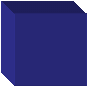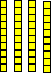The way our base 10 place value system works means that we can easily represent a number in different ways.

Let's look at the thousands column of 3145. If we record '3' in the thousands column, then we mean 3000. We could also represent 3 thousands as 30 hundreds or 300 tens or 3000 ones as shown below.

 3 thousands = 30 hundreds = 300 tens = 3000 ones 1 hundred = 10 tens = 100 ones 4 tens = 40 ones 5 ones

Can you see the pattern here? As we move a number to the next column to the left, we must multiply it by 10. If we move it to the next column to the left we must multiply it by 10 again, and so on.

Using these ideas, we can express the number 3145 in different ways;

 thousands hundreds tens ones 3 1 4 5 31 4 5 314 5 3145 31 45 3 1 45

Can you think of some more combinations?

Example 2: Express 8032 in as many different ways as you can think of.

8032 ones
803
tens and 2 ones
80 hundreds and 32 ones
8 thousand and 32 ones

We call this process renaming. It is also known as trading or regrouping. Renaming is a very important strategy when performing operations.

Models for place value

It is important to understand how the number names of our number system relate to models and number symbols so that we can talk about, record, and manipulate them.

 23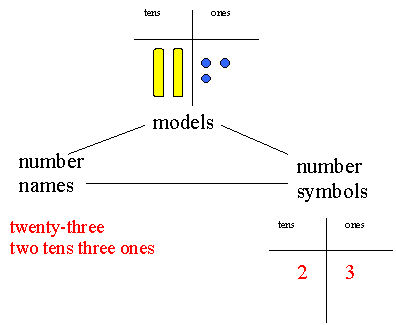As we can see above, the number 23 can be represented using materials and can be written in words and in symbols. In order for any of these representations to have meaning for us we must understand the place value system that underpins them.

MAB models

Multibase Arithmetic Blocks can be used to represent numbers. A number is represented by the total volume of the pieces used. MAB can demonstrate the concept of renaming which is crucial to performing operations.

Ten ones can be 'traded' for 1 long, because they have the same volume. This shows that 10 ones can be renamed as 1 ten.

In the picture below 20 ones is also represented by 2 longs.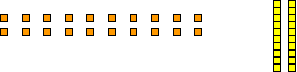A flat is made up of 10 longs, therefore 10 longs can be represented as 1 flat. This shows that 10 tens can be renamed as 1 hundred.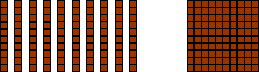By using materials such as MAB, children have the opportunity to practise renaming with concrete materials before using this concept with abstract number symbols.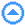Quick quiz

 Think of as many ways as you can to express the following numbers 1. 4095 2. 31736 3. 3960 4. 1212

Further questions

| Set A | Set B | Set C | Set D | Set E |

Set A:

1. Which digit is in the thousands position in the number 427 613?

2. Which digit is in the ones position in the number 635 274?

3. Which digit is in the hundreds of thousands position in the number 1 756 829?

Set B:

1. How many thousands are represented by the digit in purple type in the number 127 435?

2. How many tens of thousands are represented by the digit in purple type in the number 53 217?

3. How many tens are represented by the digit in purple type in the number 94 765?

Set C:

Use numerals to represent the following numbers.

1. Five hundreds and thirty-nine ones.

2. Nine thousands and seven ones.

3. Forty-two thousands and sixteen ones.

4. Six thousands and ninety-five ones.

5. Eight hundred and five thousands, two hundreds and twelve ones.

Set D:

Round 3 514 698 to the nearest

1. hundred

2. ten thousand

3. million

Set E:

1. To find an estimate for 89 x 29 a student mentally said 90 x 30 = 2 700

Would this answer be more or less than the exact answer to the given question? Explain why.

2. To find an estimate for 137 + 59 a student mentally said 140 + 60 = 200

Would this answer be more or less than the exact answer to the given question? Explain why.

3. To find an estimate for 52 x 16 a student mentally said 50 x 10 = 500

Would this answer be more or less than the exact answer to the given question? Explain why.

4. To find an estimate for 162 - 119 a student mentally said 160 - 120 = 40

Would this answer be more or less than the exact answer to the given question? Explain why.

5. To find an estimate for 295 + 187 a student mentally said 300 + 200 = 500

Would this answer be more or less than the exact answer to the given question? Explain why.

6. To find an estimate for 89 ÷ 31 a student mentally said 90 ÷ 30 = 3

Would this answer be more or less than the exact answer to the given question? Explain why.

7. To find an estimate for 401 x 22 a student mentally said 400 x 20 = 8,000

Would this answer be more or less than the exact answer to the given question? Explain why.